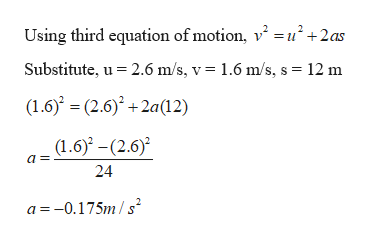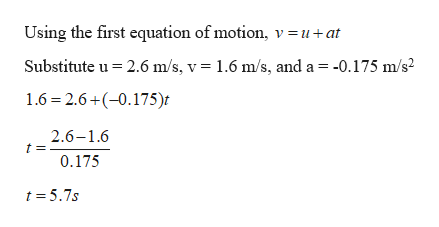# A boat is cruising in a straight line at a constant speed of 2.6 m/s when it is shifted into neutral. After coasting 12 m the engine is engaged again, and the boat resumes cruising at the reduced constant speed of 1.6 m/s. Assuming constant acceleration while coasting, (a) how much time did it take for the boat to coast the 12 m? (b) What was the boat’s acceleration while it was coasting? (c) What was the speed of the boat when it had coasted for 6.0 m? Explain.

Question
499 views

A boat is cruising in a straight line at a constant speed of 2.6 m/s when it is shifted into neutral. After coasting 12 m the engine is engaged again, and the boat resumes cruising at the reduced constant speed of 1.6 m/s. Assuming constant acceleration while coasting, (a) how much time did it take for the boat to coast the 12 m? (b) What was the boat’s acceleration while it was coasting? (c) What was the speed of the boat when it had coasted for 6.0 m? Explain.

check_circle

Step 1

Given:

Initial speed of the boat, u = 2.6 m/s

Final speed of the boat, v = 1.6 m/s

Distance travelled by the boat while coasting, s = 12 m

Step 2

(b) Calculating the acceleration of the boat while it was coasting:help_outlineImage TranscriptioncloseUsing third equation of motion, v = u2 +2as Substitute, u 2.6 m/s, v = 1.6 m/s, s = 12 m (1.6(2.6)+2a(12) (1.6) -(2.6 a = 24 -0.175m/s fullscreen
Step 3

(a) Calculating the time taken b...help_outlineImage TranscriptioncloseUsing the first equation of motion, v =u+at Substitute u 2.6 m/s, v = 1.6 m/s, and a = -0.175 m/s2 1.6 2.6+(-0.175) 2.6-1.6 0.175 t 5.7s fullscreen

### Want to see the full answer?

See Solution

#### Want to see this answer and more?

Solutions are written by subject experts who are available 24/7. Questions are typically answered within 1 hour.*

See Solution
*Response times may vary by subject and question.
Tagged in
SciencePhysics

### Kinematics